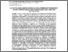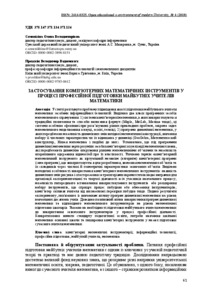# Application of computer mathematical tools in professional preparation of future teachers of mathematics

Прошкін, Володимир Вадимович (2018) Application of computer mathematical tools in professional preparation of future teachers of mathematics Open Educational E-environment of modern university (4). pp. 61-73. ISSN 2414-0325Preview Text V_Proshkin_VOSSU_4_FITU.pdf Download (817kB) | Preview

## Abstract

Abstract. The article deals with the problem of improving the quality of the future teacher of mathematics by means of information technologies. There are two classes of software tools of mathematical direction such as 1) systems of computer mathematics which use traditional notation and methods of writing formulas (Maple, MatLab, Maxima, etc.), these systems are particularly effective in solving various applications, primarily mathematical modeling tasks in science and technology; 2) programs of dynamic mathematics which provide the possibility of dynamic changes of the original mathematical design, the study of the set of its numerical characteristics or their relations in dynamics (GeoGebra, Cabri, Mathkit, and the like). It is established that under the programs of dynamic mathematics it is necessary to understand the means of computer visualization of mathematical knowledge, which provide dynamic operation of various mathematical objects and the possibility of operative obtaining information about their properties. The term "computer mathematical tool" is specified as a virtual mechanism or algorithm of a computer program, or the program itself, which is used to create or study mathematical objects or their components through the numerical and geometric characteristics of the objects themselves. The methodical features of the use of computer mathematical tools have been distinguished: the possibility of dynamic changes in the drawing and observation of the trajectories of individual points opens the way for organizing the experimental and creative activity of all participants in the educational process; the ability to pre-install the tools or to expand the toolkit that simplifies the process of building or limiting tools; the computer frees the teacher from a debilitating check of the construction, and so on. The results of the experiment related to the study of the influence of the programs of dynamic mathematics on the level of academic students’ achievements have been presented. The positive influence of the use of dynamic mathematics programs and the corresponding mathematical tools on the level of mathematical preparation of schoolchildren has been proved. The necessity of preparing the future teacher of mathematics for using this tool in the process of professional activity has been indicated. The requirements of the standard of pedagogical education, the needs of school maths in the main school and the distribution of computer tools within the framework of training a modern mathematics teacher have been specified.

Item Type: Article computer mathematical tools; information technology; professional training; future teacher; mathematics Статті у наукометричних базах > Erih PlusСтатті у журналах > Наукові (входять до інших наукометричних баз, крім перерахованих, мають ISSN, DOI, індекс цитування) Факультети > Факультет інформаційних технологій та управління > Кафедра комп'ютерних наук і математики Володимир Вадимович Прошкін 13 Dec 2018 09:57 13 Dec 2018 09:57 https://elibrary.kubg.edu.ua/id/eprint/25391View Item